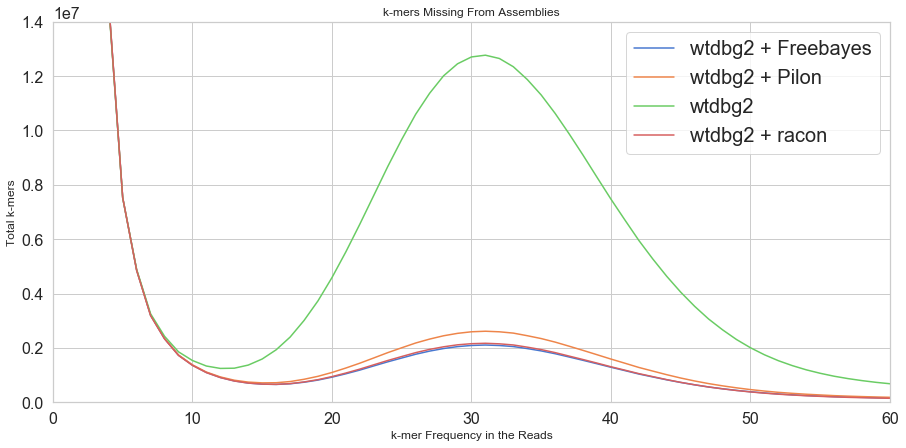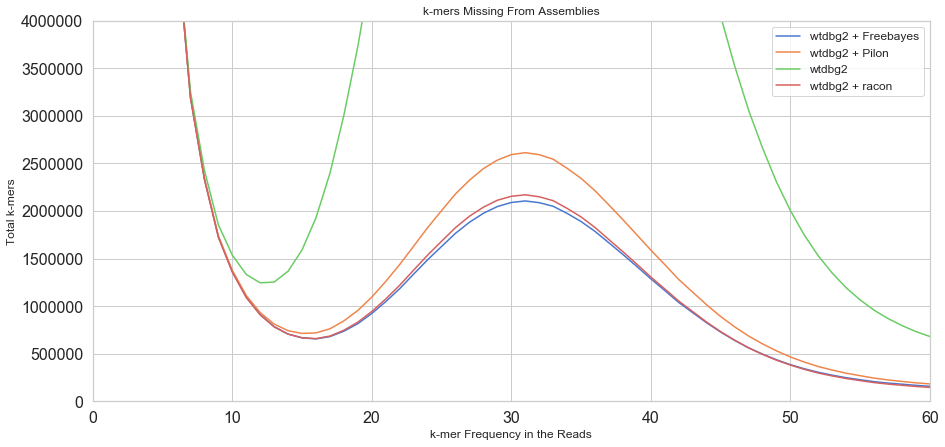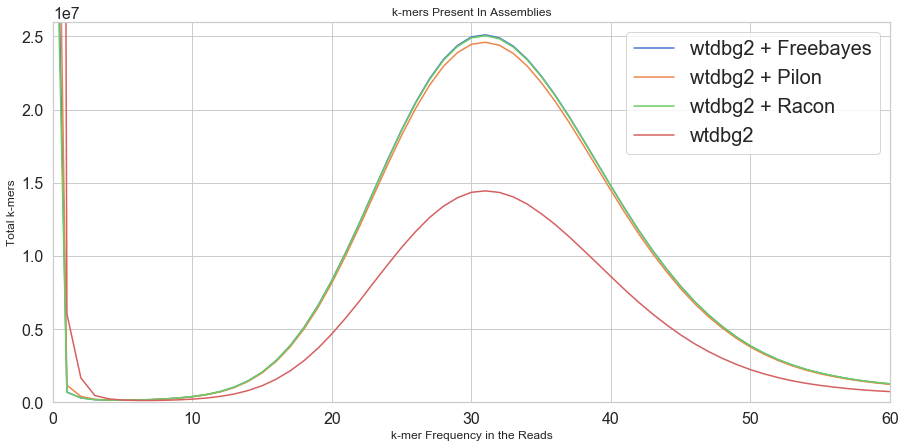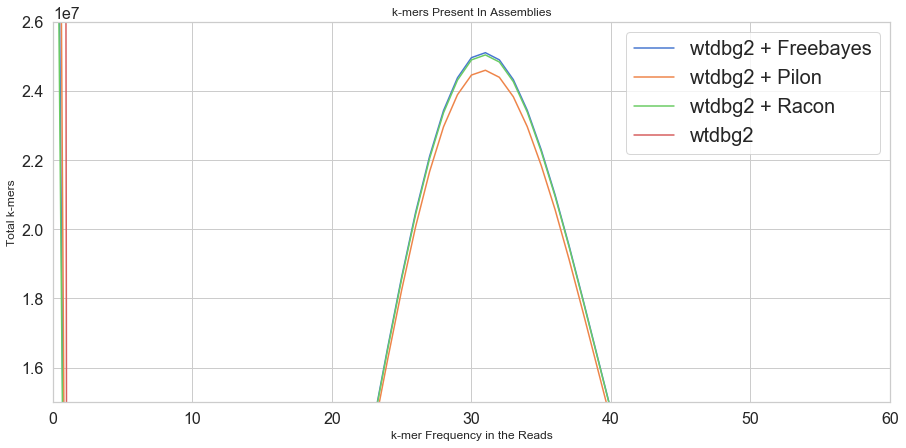Comparing Short Read Polishers

Posted on Fri 06 September 2019 in genomics

"Polishing" is the process of using short accurate Illumina sequencing reads to correct errors in noisy draft genome assemblies. Here, I will try out a few popular short-read genome assembly polishing tools/techniques and compare their efficacy by looking at assembly k-mer content. We will start with a draft genome assembly of a modern cultivated tomato line. This line was sequenced with ONT and the reads were assembled with the wtdbg2 assembler (v2.3). We will then polish this input assembly with the following polishing tools:

1. freebayes/bcftools (v1.2.0-10-g8a0dbee/v1.9)
2. Racon (v1.3.3)
3. Pilon (v1.22)

Key to understanding the success of these polishers is establishing an independent dataset to measure assembly accuracy. For this particular accession, I have randomly divided a set of Illumina sequencing reads into two distinct subsets ("polishing" and "accuracy"), each amounting to roughly 40X coverage. We feed the "polishing set" into our polishing tools in order to correct our assemblies. We can then use the "accuracy set" to measure the relative accuracy of each polished genome. I will describe our accuracy measurement once we get there. Let's get started!

In :
import pandas as pd
import numpy as np
import matplotlib.pyplot as plt
import seaborn as sns

sns.set(style="whitegrid", palette="muted", color_codes=True)
MED_FONT = 16

%matplotlib inline

Background¶

The Tomato Genome¶

This particular accession is a modern inbred Solanum lycoperiscum cultivated line. For the purposes of this notebook, it is important to note that the genome should be very homozygous. Also, the genome size is around 950 Mbp. We sequenced this accession with a combination of MinION and GridION flowcells.

The assembly¶

For those who are interested, the assembly was produced with the following commands:

wtdbg2 -f -t -g 950m -L 16873 -x ont -i reads.fasta -o asm
wtpoa-cns -i asm.ctg.lay.gz -fo asm.ctg.fasta
minimap2 -ax map-ont asm.ctg.fasta reads.fasta > alns.sam
samtools view -bS alns.sam -o alns.bam
samtools sort -@ 4 alns.bam -o alns.sorted.bam
samtools view alns.sorted.bam -o alns.sorted.sam
wtpoa-cns -d asm.ctg.fasta -i alns.sorted.sam -fo asm.ctg.cns.fasta

We can see that this assembly went through an extra consensus round with wtpoa-cns. This yielded an N50 of 1,408,448 bp and a total assembly size of 731,724,146 bp.

Polishing¶

As stated before, we polished this wtdbg2 assembly with freebayes/bcftools, Pilon and Racon. The assembly was polished with our "polishing set" of Illumina reads, which were aligned to our assembly with bowtie2 using the following commands:

bowtie2 --local -p 8 -x asm.ctg.cns.fasta -U trimmed.both.renamed.fastq -S alns.sam

Freebayes/bcftools polishing was run with the same parameters as described in the VGP GitHub repo. For pilon, I used the "--fix bases" and "--diploid" flags. Finally, I ran Racon with default parameters (including "-u").

Polishing Performance Comparison¶

Finally, we get to the good part: comparing the polished assemblies to see which polisher performed best. For this, we will use KAT. Given our independent "accuracy set" of Illumina reads, KAT will establish a database of k-mers. We can then compare k-mers in our assemblies with k-mers in our short reads to observe general trends in the assembly.

Using kat comp, we establish the following matrix for each of our polished assemblies.

Row index $i$ = k-mer frequency in the reads

Column index $j$ = k-mer frequency in the assembly

$e_{i, j} =$ the number of distinct k-mers with frequency $i$ in the reads and frequency $j$ in the assembly.

With this matrix, we can do cool things like look for k-mers in the assembly to that do not appear in the short reads. Or we can look for k-mers in the short reads that do not appear in the assembly. Let's take a closer look.

In :
df_fb = pd.read_csv("fb_kat_comp-main.mx", sep=" ", skiprows=12, names=["V" + str(i) for i in range(1001)])
df_pi = pd.read_csv("pi_kat_comp-main.mx", sep=" ", skiprows=12, names=["V" + str(i) for i in range(1001)])
df_ra = pd.read_csv("ra_kat_comp-main.mx", sep=" ", skiprows=12, names=["V" + str(i) for i in range(1001)])
df_wt = pd.read_csv("wtdbg2_no_polish_comp-main.mx", sep=" ", skiprows=12, names=["V" + str(i) for i in range(1001)])

Missing k-mers¶

First, we will check how (likely) true k-mers are missing from the assembly.

In :
plt.figure(figsize=(15,7))
plt.plot(df_fb["V0"], label="wtdbg2 + Freebayes")
plt.plot(df_pi["V0"], label="wtdbg2 + Pilon")
plt.plot(df_wt["V0"], label="wtdbg2")
plt.plot(df_ra["V0"], label="wtdbg2 + racon")
plt.xlim(0,60)
plt.ylim(0,14000000)
plt.legend(prop={'size': 20})
plt.rc('xtick', labelsize=MED_FONT)
plt.rc('ytick', labelsize=MED_FONT)
plt.xlabel("k-mer Frequency in the Reads")
plt.ylabel("Total k-mers")
plt.title("k-mers Missing From Assemblies")
plt.show()To find the number of assembly k-mers that are missing from the reads, all we have to do is plot the first column of our matrix. Remember that the first column ( j=0 ) refers to k-mers that appear with frequency=0 in the assembly. Then, each element index in this vector will correspond to a frequency in the reads. Above, we plot this vector for each assembly. We also note that, given these curves, we can informally say that k-mers in the reads with frequency less than ~15 are probably error k-mers. However, k-mers that have a frequency >= 15 are expected to be present in an accurate assembled representation of our genome.

Firstly, we see that our unpolished assembly is missing the most k-mers. That is expected since the unpolished assembly will have the most assembly errors, and those errors will yield incorrect k-mers (k-mers that span the error) in place of correct k-mers. Next, we see that, of the polished assemblies, our Pilon assembly is missing the most k-mers. Finally, it is close, but the Racon assembly is missing a few more k-mers than the Freebayes assembly. Let's make the same plot, but zoom in again.

In :
plt.figure(figsize=(15,7))
plt.plot(df_fb["V0"], label="wtdbg2 + Freebayes")
plt.plot(df_pi["V0"], label="wtdbg2 + Pilon")
plt.plot(df_wt["V0"], label="wtdbg2")
plt.plot(df_ra["V0"], label="wtdbg2 + racon")
plt.xlim(0,60)
plt.ylim(0,4000000)
plt.legend(prop={'size': 12})
plt.rc('xtick', labelsize=MED_FONT)
plt.rc('ytick', labelsize=MED_FONT)
plt.xlabel("k-mer Frequency in the Reads")
plt.ylabel("Total k-mers")
plt.title("k-mers Missing From Assemblies")
plt.show()Present k-mers¶

Similarly, we can observe how many k-mers in each assembly are present in the short-reads k-mers. For this, we just sum columns 1 through n, since we don't care how frequent a k-mer is in the assembly.

In :
plt.figure(figsize=(15,7))
plt.plot(np.sum(df_fb.values[:, 1:], axis=1), label="wtdbg2 + Freebayes")
plt.plot(np.sum(df_pi.values[:, 1:], axis=1), label="wtdbg2 + Pilon")
plt.plot(np.sum(df_ra.values[:, 1:], axis=1), label="wtdbg2 + Racon")
plt.plot(np.sum(df_wt.values[:, 1:], axis=1), label="wtdbg2")
plt.xlim(0,60)
plt.ylim(0,26000000)
plt.rc('xtick', labelsize=MED_FONT)
plt.rc('ytick', labelsize=MED_FONT)
plt.legend(prop={'size': 20})
plt.xlabel("k-mer Frequency in the Reads")
plt.ylabel("Total k-mers")
plt.title("k-mers Present In Assemblies")
plt.show()With regards to relative performance, the tools rank the same as before. In our unpolished assemblies, we have the fewest assembly k-mers present in the short-read k-mer database, whereas our Freebayes polished assembly has the most k-mers present in the short-read k-mer database. Let's zoom in again.

In :
plt.figure(figsize=(15,7))
plt.plot(np.sum(df_fb.values[:, 1:], axis=1), label="wtdbg2 + Freebayes")
plt.plot(np.sum(df_pi.values[:, 1:], axis=1), label="wtdbg2 + Pilon")
plt.plot(np.sum(df_ra.values[:, 1:], axis=1), label="wtdbg2 + Racon")
plt.plot(np.sum(df_wt.values[:, 1:], axis=1), label="wtdbg2")
plt.xlim(0,60)
plt.ylim(15000000,26000000)
plt.rc('xtick', labelsize=MED_FONT)
plt.rc('ytick', labelsize=MED_FONT)
plt.legend(prop={'size': 20})
plt.xlabel("k-mer Frequency in the Reads")
plt.ylabel("Total k-mers")
plt.title("k-mers Present In Assemblies")
plt.show()Conclusions¶

In this simple analysis, it appears that Racon and Freebayes/bcftools are superior polishers to Pilon. We also see that Freebayes/bcftools has the slight edge over-all. Ultimately, I like this consensus accuracy analysis because it is very explicit. From short-reads, we can establish a database of accurate k-mers that we expect to find in our assembly. Therefore, measuring how many of those k-mers are missing from an assembly or how many correct k-mers are present in an assembly is a nice way to assess accuracy. One challenge with this technique is that one needs enough data to hold out an independent validation set of short-reads.

Of course, this analysis does not address many of the subtleties of polishing. Firstly, alignment of short-reads can have a huge effect on polishing. What would have happened if I had used bwa mem instead of bowtie2? And how about parameter adjustment or alignment filtering? Also, how would these polishers perform given multiple rounds of polishing? And finally, this analysis doesn't address specific questions about the nature of polishing for each tool. How do the tools polish regions with lower vs. higher coverage? How do these tools polish different types of errors?

Regardless, I think I will be sticking with Freebayes/bcftools in the future :)